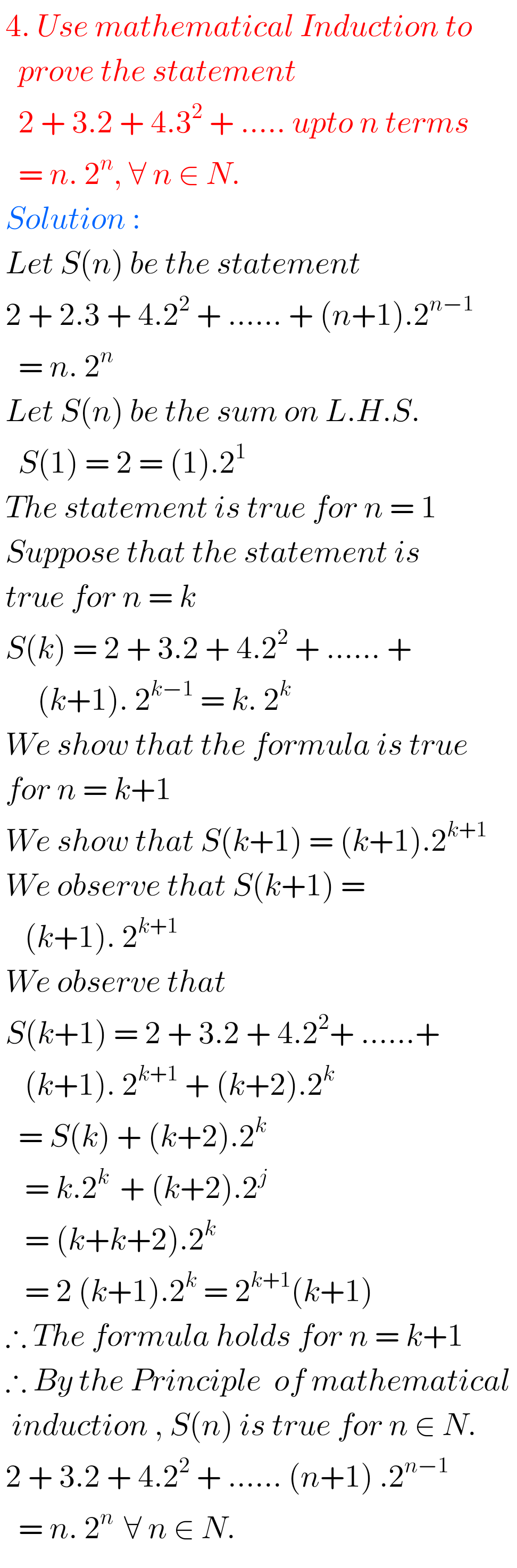## MATHEMATICAL INDUCTION, Intermediate 1st year problems with solutions,solutions for Mathematical Induction Inter first year 1A

MATHEMATICAL INDUCTION, INTERMEDIATE FIRST YEAR PROBLEMS WITH SOLUTIONS Mathematics Intermediate first year 1A Mathematical Induction solutions for some problems.  These solutions are very simple to understand.  Study the textbook lesson Mathematical Induction very well  Observe the example problems and solutions given in the textbook  You can also see Inter Maths 1A textbook solutions Inter Maths …